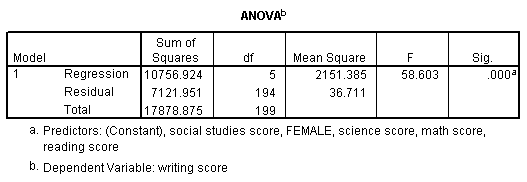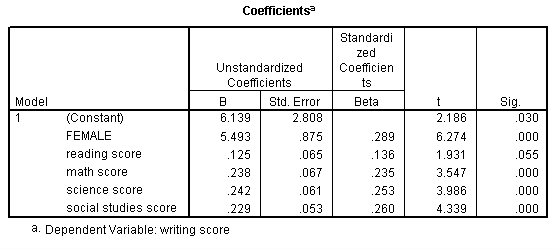## One sample t-test

Multiple regression is very similar to simple regression, except that in multiple regression you have more than one predictor variable in the equation. For example, using the hsb2 data file we will predict writing score from gender (female), reading, math, science and social studies (socst) scores.

regression variable = write female read math science socst /dependent = write /method = enter.The results indicate that the overall model is statistically significant (F = 58.60, p = 0.000). Furthermore, all of the predictor variables are statistically significant except for read.